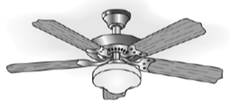Chapter 6.1, Problem 27E### Elementary Geometry for College St...

6th Edition
Daniel C. Alexander + 1 other
ISBN: 9781285195698

#### Solutions

Chapter
Section### Elementary Geometry for College St...

6th Edition
Daniel C. Alexander + 1 other
ISBN: 9781285195698
Textbook Problem
1 views

# A ceiling fan has equally spaced blades. What is the measure of the angle formed by two consecutive blades if there are a) 5 blades? b) 6 blades?To determine

(a)

To Find: The measure of the angle formed by two consecutive blades if there are 5 blades.

Explanation

Given:

A ceiling are equally spaced 5 blades.

Postulate used:

Central angle postulate:

In a circle, the degree measure of a central angle is equal to the degree measure of its intercepted arc.

Congruent arcs:

In a circle, congruent arcs are arcs with equal measures.

Since, the sum of the measures of the consecutive arc that form a circle is 360o.

Calculation:

Consider, the figure given below:

Since, the 5 blades are equally spaced.

Therefore, in a circle, congruent arcs are arcs with equal measures.

mAB=mBC

mBC=mCD

mCD=mDE

And

mDE=mEA

Since, the sum of the measures of the consecutive arc that form a circle is 360o.

mAB+mBC+mCD+mDE+mEA=360o

By using the above result we obtain

To determine

(b)

To calculate:

The measure of the angle formed by two consecutive blades if there are 6 blades.

### Still sussing out bartleby?

Check out a sample textbook solution.

See a sample solution

#### The Solution to Your Study Problems

Bartleby provides explanations to thousands of textbook problems written by our experts, many with advanced degrees!

Get Started

#### Simplify: 1236

Elementary Technical Mathematics

#### Evaluate the expression sin Exercises 116. 323

Finite Mathematics and Applied Calculus (MindTap Course List)

#### By definition the improper integral It is not an improper integral.

Study Guide for Stewart's Single Variable Calculus: Early Transcendentals, 8th

#### True or False: These lines are skew:

Study Guide for Stewart's Multivariable Calculus, 8th